# Vector product

A vector product, also known as cross product, is an antisymmetric product A×B = −B×A of two vectors A and B in 3-dimensional Euclidean space ℝ3. The vector product is again a 3-dimensional vector. The vector product is widely used in many areas of mathematics, mechanics, electromagnetism, gravitational fields, etc.

A proper vector changes sign under inversion, while a cross product is invariant under inversion [both factors of the cross product change sign and (−1)×(−1) = 1]. A vector that does not change sign under inversion is called an axial vector or pseudo vector. Hence a cross product is a pseudo vector. A vector that does change sign is often referred to as a polar vector in this context.

## Contents

###  Definition

Given two vectors, A and B in ℝ3, the vector product is a vector with length AB sin θ where A is the length of A, B is the length of B, and θ is the smaller (non-reentrant) angle between A and B. The direction of the vector product is perpendicular (or normal) to the plane containing the vectors A and B and follows the right-hand rule,$\mathbf{A}\times \mathbf{B} = \hat{\mathbf{n}}\, |\mathbf{A}|\, |\mathbf{B}|\,\sin\theta,$

where$\hat{\mathbf{n}}$ is a unit vector normal to the plane spanned by A and B in the right-hand rule direction, see Fig. 1 (in which vectors are indicated by lowercase letters).

We recall that the length of a vector is the square root of the dot product of a vector with itself, A ≡ |A| = (A⋅A )1/2 and similarly for the length of B. A unit vector has by definition length one.

From the antisymmetry A×B = −B×A follows that the cross (vector) product of any vector with itself (or another parallel or antiparallel vector) is zero because A×A = − A×A and the only quantity equal to minus itself is the zero. Alternatively, one may derive this from the fact that sin(0) = 0 (parallel vectors) and sin(180) = 0 (antiparallel vectors).

##  The right hand rule

The diagram in Fig. 1 illustrates the direction of a×b, which follows the right-hand rule. If one points the fingers of the right hand towards the head of vector a (with the wrist at the origin), then moves the right hand towards the direction of b, the extended thumb will point in the direction of a×b. If a and b are interchanged the thumb will point downward and the cross product has the opposite sign.

## Definition with the use of the Levi-Civita symbol

A concise definition of the vector product is by the use of the antisymmetric Levi-Civita symbol,$\epsilon_{k\ell m} = \begin{cases} 0 & \hbox{if two or more indices are zero} \\ 1 & \hbox{if }k \ell m \hbox{ is an even permutation of } x y z \\ -1 & \hbox{if }k \ell m \hbox{ is an odd permutation of } x y z. \\ \end{cases}$

Examples,$\epsilon_{x,y,z} = \epsilon_{z, x,y} = 1,\quad \epsilon_{y,x,z} = -1,\quad \epsilon_{x,y,y} =0.$

In terms of the Levi-Civita symbol the component i of the vector product is defined as,$A_i = (\mathbf{B}\times\mathbf{C})_i \quad\Longleftrightarrow \quad A_i = \epsilon_{ijk} B_j C_k,$

where summation convention is implied. That is, repeated indices are summed over (in this case sum is over j and k).

##  Another formulation of the cross product

Rather than in terms of angle and perpendicular unit vector, another form of the cross product is often used. In the alternate definition the vectors must be expressed with respect to a Cartesian (orthonormal) coordinate frame ax, ay and az of ℝ3.

With respect to this frame we write A = (Ax, Ay, Az) and B = (Bx, By, Bz). Then

A×B = (AyBz - AzBy) ax + (AzBx - AxBz) ay + (AxBy - AyBx) az.

This formula can be written more concisely upon introduction of a determinant:$\mathbf A \times \mathbf B = \left|\begin{array}{ccc} \mathbf a_x & \mathbf a_y & \mathbf a_z \\ A_x & A_y & A_z \\ B_x & B_y & B_z \end{array} \right|,$

where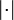$\left|\cdot\right|$ denotes the determinant of a matrix. This determinant must be evaluated along the first row, otherwise the equation does not make sense.

## Geometric representation of the lengthFig. 2. The length of the cross product A×B is equal to area of the parallelogram with sides a and b, the sum of the areas of the dotted and dashed triangle.

The length of the cross product of vectors A and B is equal to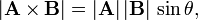$|\mathbf{A}\times \mathbf{B}| = |\mathbf{A}|\, |\mathbf{B}|\,\sin\theta,$

because$\hat{\mathbf{n}}$ has by definition length 1. Using the high school geometry rule: the area S of a triangle is its base a times its half-height d, we see in Fig. 2 that the area S of the dotted triangle is equal to: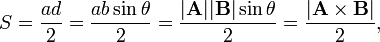$S = \frac{ad}{2} = \frac{ab\sin\theta}{2}= \frac{|\mathbf{A}||\mathbf{B}|\sin\theta}{2} = \frac{|\mathbf{A}\times \mathbf{B}|}{2},$

because, as follows from Fig. 2: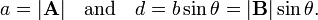$a = |\mathbf{A}| \quad\textrm{and}\quad d = b \sin\theta = |\mathbf{B}|\sin\theta .$

Hence |A×B| = 2S. Since the dotted triangle with sides a, b, and c is congruent to the dashed triangle, the area of the dotted triangle is equal to the area of the dashed triangle and the length 2S of the cross product is equal to the sum of the areas of the dotted and the dashed triangle.

In conclusion:

The length (magnitude) of A×B is equal to the area of the parallelogram spanned by the vectors A and B.

### Application: the volume of a parallelepiped

The volume V of the parallelepiped shown in Fig. 3 is given by$V = \mathbf{A}\cdot (\mathbf{B}\times\mathbf{C}).$

Indeed, remember that the volume of a parallelepiped is given by the area S of its base times its height h, V = Sh. Above it was shown that, if$\mathbf{D} \equiv \mathbf{B}\times\mathbf{C}\quad\textrm{then}\quad S = |\mathbf{D}|.$

The height h of the parallelepiped is the length of the projection of the vector A onto D. The dot product between A and D is |D| times the length h,$\mathbf{D}\cdot\mathbf{A}= |\mathbf{D}|\,\big(|\mathbf{A}| \cos\phi\big) = |\mathbf{D}|\, h = S h,$

so that V = (B×C)⋅A = A⋅(B×C).

It is of interest to point out that V can be given by a determinant that contains the components of A, B, and C with respect to a Cartesian coordinate system,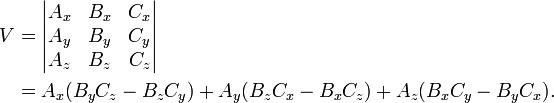\begin{align} V &= \begin{vmatrix} A_x & B_x & C_x \\ A_y & B_y & C_y \\ A_z & B_z & C_z \\ \end{vmatrix} \\ &= A_x (B_y C_z - B_z C_y) + A_y(B_z C_x - B_x C_z) + A_z(B_x C_y - B_y C_x). \end{align}

From the permutation properties of a determinant it follows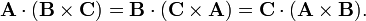$\mathbf{A}\cdot (\mathbf{B}\times\mathbf{C}) = \mathbf{B}\cdot (\mathbf{C}\times\mathbf{A}) =\mathbf{C}\cdot (\mathbf{A}\times\mathbf{B}).$

The product A⋅(B×C) is often referred to as a triple scalar product. It is a pseudo scalar. The term scalar refers to the fact that the triple product is invariant under the same simultaneous rotation of A, B, and C. The term pseudo refers to the fact that simultaneous inversion

A → −A, B → −B, and C → −C

converts the triple product into minus itself, while a proper scalar is invariant under inversion.

## Cross product as linear map

Given a fixed vector n, the application of n× is linear,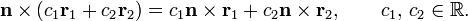$\mathbf{n}\times( c_1 \mathbf{r}_1 + c_2 \mathbf{r}_2) = c_1 \mathbf{n}\times \mathbf{r}_1 + c_2 \mathbf{n}\times \mathbf{r}_2, \qquad c_1,\, c_2 \in \mathbb{R}.$

This implies that n×r can be written as a matrix-vector product,$\mathbf{n}\times \mathbf{r} = \begin{pmatrix} n_y r_z - n_z r_y \\ n_z r_x - n_x r_z \\ n_x r_y - n_y r_x \end{pmatrix} = \underbrace{ \begin{pmatrix} 0 & -n_z & n_y \\ n_z& 0 & -n_x \\ -n_y& n_x & 0 \end{pmatrix}}_{\mathbf{N}} \begin{pmatrix} r_x \\ r_y \\ r_z \end{pmatrix} = \mathbf{N}\, \mathbf{r}.$

The matrix N has as general element$N_{\alpha \beta} = -\sum_{\gamma=x,y,z} \epsilon_{\alpha \beta \gamma} n_\gamma \,$

where εαβγ is the antisymmetric Levi-Civita symbol. It follows that$\left( \mathbf{n}\times \mathbf{r} \right)_\alpha = \sum_{\beta=x,y,z} N_{\alpha\beta}\; r_\beta = - \sum_{\beta=x,y,z}\sum_{\gamma=x,y,z} \epsilon_{\alpha \beta \gamma} n_\gamma r_\beta = \sum_{\beta=x,y,z}\sum_{\gamma=x,y,z} \epsilon_{\alpha \beta \gamma} n_\beta r_\gamma.$

## Relation to infinitesimal rotation

The rotation of a vector r around the unit vector$\hat{\mathbf{n}}$ over an angle φ sends r to r′. The rotated vector is related to the original vector by (see this article for a proof):$\mathbf{r}' = \mathbf{r}\;\cos\phi + \hat{\mathbf{n}}\;\big(\hat{\mathbf{n}}\cdot \mathbf{r}\big)\big(1-\cos\phi\big) + (\hat{\mathbf{n}}\times\mathbf{r} )\;\sin\phi.$

Suppose now that φ is infinitesimal and equal to Δφ, i.e., squares and higher powers of Δφ are negligible with respect to Δφ, then$\cos\Delta \phi = 1,\quad\hbox{and}\quad \sin\Delta\phi = \Delta\phi$

so that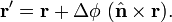$\mathbf{r}' = \mathbf{r} + \Delta\phi\;(\hat{\mathbf{n}}\times\mathbf{r} ) .$

The linear operator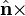$\hat{\mathbf{n}}\times$  maps   ℝ3→ℝ3 ; it is known as the generator of an infinitesimal rotation around$\hat{\mathbf{n}}$.

## Triple product

It holds that\begin{align} \mathbf{A}\times(\mathbf{B}\times\mathbf{C}) &= \mathbf{B}(\mathbf{A}\cdot\mathbf{C}) - \mathbf{C}(\mathbf{A}\cdot\mathbf{B})\\ (\mathbf{A}\times\mathbf{B})\times\mathbf{C} &= \mathbf{B}(\mathbf{A}\cdot\mathbf{C}) - \mathbf{A}(\mathbf{B}\cdot\mathbf{C})\\ \end{align}

The proof is straigthforward. Upon writing the vectors in terms of a Cartesian basis ax, ay, az the first step is,$\mathbf{A}\times(\mathbf{B}\times\mathbf{C}) = \Big(A_x \mathbf{a}_x +A_y \mathbf{a}_y+ A_z \mathbf{a}_z\Big)\times \Big( (B_yC_z - B_zC_y) \mathbf{a}_x + (B_zC_x - B_xC_z) \mathbf{a}_y + (B_xC_y - B_yC_x) \mathbf{a}_z \Big)$

The second step is\begin{align} \mathbf{A}\times(\mathbf{B}\times\mathbf{C}) &= \Big( A_y(B_xC_y - B_yC_x) - A_z(B_zC_x - B_xC_z) \Big) \mathbf{a}_x \\ & + \Big( A_z(B_yC_z - B_zC_y) - A_x(B_xC_y - B_yC_x) \Big) \mathbf{a}_y \\ & + \Big( A_x(B_zC_x - B_xC_z) - A_y(B_yC_z - B_zC_y) \Big) \mathbf{a}_z \\ \end{align}

On the other hand\begin{align} \mathbf{B}(\mathbf{A}\cdot\mathbf{C})- \mathbf{C}(\mathbf{A}\cdot\mathbf{B}) &= \Big( B_x(A_xC_x+A_yC_y+A_zC_z) - C_x (A_xB_x+A_yB_y+A_zB_z)\Big) \mathbf{a}_x \\ &+\Big( B_y(A_xC_x+A_yC_y+A_zC_z) - C_y (A_xB_x+A_yB_y+A_zB_z)\Big) \mathbf{a}_y \\ &+\Big( B_z(A_xC_x+A_yC_y+A_zC_z) - C_z (A_xB_x+A_yB_y+A_zB_z)\Big) \mathbf{a}_z \\ &= \Big( (A_y B_x C_y+A_z B_x C_z) - (A_y B_y C_x+A_z B_z C_x)\Big) \mathbf{a}_x \\ &+\Big( (A_x B_y C_x+A_z B_y C_z) - (A_x B_x C_y+A_z B_z C_y)\Big) \mathbf{a}_y \\ &+\Big( (A_x B_z C_x+A_y B_z C_y) - (A_x B_x C_z+A_y B_y C_z)\Big) \mathbf{a}_z \\ \end{align}

Comparison of the coefficients of the basis vectors proves the equality of the first triple product. Obviously the proof of the second triple product runs along the same lines.

A more elegant proof is with the aid of the antisymmetric Levi-Civita symbol, that satisfies the following property (summation convention is implied, that is, repeated indices are summed over):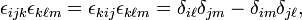$\epsilon_{ijk}\epsilon_{k\ell m} = \epsilon_{kij}\epsilon_{k\ell m}= \delta_{i\ell}\delta_{j m} - \delta_{im}\delta_{j\ell},$

where δij is a Kronecker delta,$\delta_{ij}= \begin{cases} &1\quad\text{if}\quad i = j \\ &0\quad\text{if}\quad i\ne j. \end{cases}$

Consider$\Big(\mathbf{A}\times(\mathbf{B}\times\mathbf{C})\Big)_{i} = \epsilon_{ijk}\epsilon_{k\ell m}A_jB_\ell C_m = (\delta_{i\ell}\delta_{j m} - \delta_{im}\delta_{j\ell}) A_jB_\ell C_m = A_jB_iC_j - A_jB_jC_i = (\mathbf{A}\cdot\mathbf{C})B_i - (\mathbf{A}\cdot\mathbf{B})C_i,$

which again gives the result for the first triple product.

The triple product satisfies the Jacobi identity$\mathbf{A}\times(\mathbf{B}\times\mathbf{C}) + \mathbf{B}\times(\mathbf{C}\times\mathbf{A}) + \mathbf{C}\times(\mathbf{A}\times\mathbf{B}) = \mathbf{0}$

This is proved by adding the following relations\begin{align} \mathbf{A}\times(\mathbf{B}\times\mathbf{C}) &= \mathbf{B}(\mathbf{A}\cdot\mathbf{C}) - \mathbf{C}(\mathbf{A}\cdot\mathbf{B})\\ \mathbf{B}\times(\mathbf{C}\times\mathbf{A}) &= \mathbf{C}(\mathbf{B}\cdot\mathbf{A}) - \mathbf{A}(\mathbf{B}\cdot\mathbf{C})\\ \mathbf{C}\times(\mathbf{A}\times\mathbf{B}) &= \mathbf{A}(\mathbf{C}\cdot\mathbf{B}) - \mathbf{B}(\mathbf{C}\cdot\mathbf{A})\\ \end{align}

and using AB = BA, etc.

## Generalization

### Twofold wedge product

From a somewhat more abstract point of view one may define the vector product as an element of the antisymmetric subspace of the 9-dimensional tensor product space3⊗ℝ3. This antisymmetric subspace is of dimension 3. An element of the space is sometimes written as a wedge product,$\mathbf{A}\wedge\mathbf{B} := \mathbf{A}\otimes\mathbf{B} - \mathbf{B}\otimes\mathbf{A}, \quad\hbox{with}\quad \mathbf{A},\; \mathbf{B} \in \mathbb{R}^3.$

If a1, a2. a3 is an orthonormal basis of ℝ3 and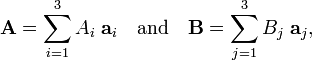$\mathbf{A} = \sum_{i=1}^3 A_i\; \mathbf{a}_i \quad \hbox{and}\quad \mathbf{B} = \sum_{j=1}^3 B_j\; \mathbf{a}_j ,$

then upon noting that$\mathbf{a}_i \wedge\mathbf{a}_j = - \mathbf{a}_j \wedge\mathbf{a}_i, \qquad \mathbf{a}_i \wedge\mathbf{a}_i = 0$

it follows that\begin{align} \mathbf{A}\wedge\mathbf{B} &= \left(\sum_{i=1}^{3} A_i \mathbf{a}_i \right) \wedge \left(\sum_{j=1}^{3} B_j \mathbf{a}_j \right) = \sum_{i,j=1}^3 A_i B_j \; (\mathbf{a}_i \wedge\mathbf{a}_j) \\ &= \sum_{ij} A_iB_j (\mathbf{a}_i \wedge\mathbf{a}_j) \\ &= \sum_{i

The basis elements are orthogonal (and easily normalized)$(\mathbf{a}_i \wedge\mathbf{a}_j) \cdot (\mathbf{a}_k \wedge\mathbf{a}_\ell) = (\mathbf{a}_i \otimes \mathbf{a}_j - \mathbf{a}_j \otimes \mathbf{a}_i) \cdot (\mathbf{a}_k \otimes \mathbf{a}_\ell - \mathbf{a}_\ell \otimes \mathbf{a}_k) =2\delta_{ik}\delta_{j\ell} - 2\delta_{i\ell}\delta_{jk}.$

Make the identification:$\sqrt{\tfrac{1}{2}}\;(\mathbf{a}_1 \wedge\mathbf{a}_2)\; \leftrightarrow\; \mathbf{e}_3, \qquad \sqrt{\tfrac{1}{2}}\;(\mathbf{a}_1 \wedge\mathbf{a}_3)\; \leftrightarrow\; -\mathbf{e}_2, \qquad \sqrt{\tfrac{1}{2}}\;(\mathbf{a}_2 \wedge\mathbf{a}_3)\; \leftrightarrow\; \mathbf{e}_1,$

and it follows that the new vectors form an orthonormal basis,$\mathbf{e}_1 \cdot\mathbf{e}_2 = \mathbf{e}_1 \cdot\mathbf{e}_3 = \mathbf{e}_2 \cdot\mathbf{e}_3 = 0, \qquad \mathbf{e}_i \cdot\mathbf{e}_i = 1, \quad i=1,2,3.$

The wedge product corresponds to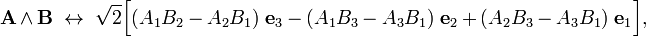$\mathbf{A}\wedge\mathbf{B}\; \leftrightarrow \; \sqrt{2}\Big[ (A_1B_2 - A_2B_1)\; \mathbf{e}_3 - (A_1B_3 - A_3B_1)\; \mathbf{e}_2 + (A_2B_3 - A_3B_1)\; \mathbf{e}_1 \Big],$

and it is concluded that cross product can be identified with the wedge product (up to the factor √2)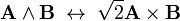$\mathbf{A}\wedge\mathbf{B} \; \leftrightarrow \; \sqrt{2} \mathbf{A}\times \mathbf{B}$

### Threefold wedge product

The association between wedge product and vector product does not hold in the case of 3-vectors (members of three-dimensional spaces) for more than two factors. Above it is shown how to expand the 3-vector A×(B×C) in terms of B and C (3-vectors), while the wedge product is the antisymmetrized projection of ABC: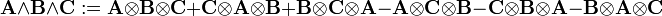$\mathbf{A}\wedge\mathbf{B}\wedge\mathbf{C} := \mathbf{A}\otimes\mathbf{B}\otimes\mathbf{C} +\mathbf{C}\otimes\mathbf{A}\otimes\mathbf{B} +\mathbf{B}\otimes\mathbf{C}\otimes\mathbf{A} -\mathbf{A}\otimes\mathbf{C}\otimes\mathbf{B} -\mathbf{C}\otimes\mathbf{B}\otimes\mathbf{A} -\mathbf{B}\otimes\mathbf{A}\otimes\mathbf{C}$

This product is non-vanishing if and only if A, B, and C are linearly independent (hence a fourfold wedge product of 3-vectors vanishes). Furthermore, if A is multiplied by the 3×3 matrix F, A′ = FA, and B′ and C′ are defined also by multiplication with F, then$\mathbf{A}'\wedge\mathbf{B}'\wedge\mathbf{C}' = \det(\mathbf{F})\; \mathbf{A}\wedge\mathbf{B}\wedge\mathbf{C},$

where det(F) is the determinant of the matrix F. If the determinant is equal to unity (as for proper rotation matrices) the threefold wedge product of 3-vectors is invariant, a scalar.

### General wedge product

In general the antisymmetric subspace of the k-fold tensor power (k-fold tensor product of the same n-dimensional space, kn):$\underbrace{\mathbb{R}^n \otimes \mathbb{R}^n\otimes \cdots\otimes\mathbb{R}^n} _{k\; \mathrm{factors}}$

is of dimension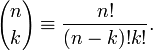${n \choose k}\equiv \frac{n!}{(n-k)!k!}.$

Elements of the antisymmetric subspace of tensor power are wedge or exterior products written as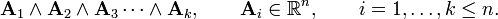$\mathbf{A}_1 \wedge \mathbf{A}_2 \wedge \mathbf{A}_3 \cdots \wedge \mathbf{A}_k,\qquad \mathbf{A}_i \in \mathbb{R}^n, \qquad i=1,\dots,k \le n.$

The antisymmetric products may be projected from the tensor power by means of the antisymmetrizer. The antisymmetric subspace of a two-fold tensor product space is of dimension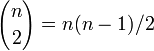${n \choose 2} = n(n-1)/2$.

The latter number is equal to 3 only if n = 3. For instance, for n = 2 or 4, the antisymmetric subspaces are of dimension 1 and 6, respectively.

It can be shown that a wedge product consisting of n−1 factors transforms as a vector in ℝn. In that sense the antisymmetric product Bk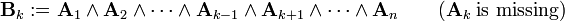$\mathbf{B}_k := \mathbf{A}_1 \wedge \mathbf{A}_2 \wedge\cdots\wedge\mathbf{A}_{k-1}\wedge\mathbf{A}_{k+1}\wedge \cdots \wedge \mathbf{A}_{n} \qquad (\mathbf{A}_{k}\;\hbox{is missing})$

is the generalization of a vector product. Clearly Bk belongs to a space of dimension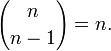${n \choose n-1} = n.$

In the case of n−1 factors one can make the association between wedge products and vectors, in the same way as for the n = 3 vector product. The n-dimensional space spanned by the wedge products Bk (k =1,2, ..., n) is the dual of ℝn.

## Note

1. In many-fermion physics a vector Ak corresponds to a fermionic particle in orbital k and Bk corresponds to a hole in orbital k; holes and particles transform contragrediently.